Next: Stokes Constants Up: Wave Propagation in Inhomogeneous Previous: Asymptotic Series

# WKB Solution as Asymptotic Series

We have seen that the WKB solution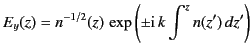(1164)

is an approximate solution of the differential equation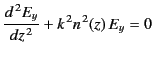(1165)

in the limit that the typical wavelength,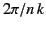, is much smaller than the typical variation length-scale of the refractive index. But, what sort of approximation is involved in writing this solution?

It is convenient to define the scaled variable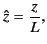(1166)

where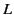is the typical variation length-scale of the refractive index,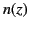. Equation (1167) can then be written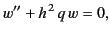(1167)

where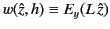,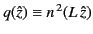,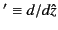, and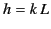. Note that, in general,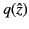,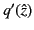,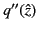, et cetera, are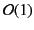quantities. The non-dimensional constant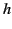is of order the ratio of the variation length-scale of the refractive index to the wavelength. Let us seek the solutions to Equation (1169) in the limit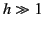.

We can write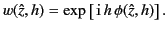(1168)

Equation (1169) transforms to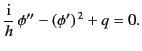(1169)

Expanding in powers of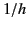, we obtain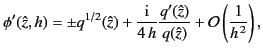(1170)

which yields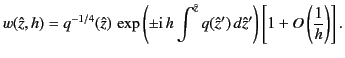(1171)

Of course, we immediately recognize this expression as a WKB solution.

Suppose that we keep expanding in powers ofin Equation (1172). The appropriate generalization of Equation (1173) is a series solution of the form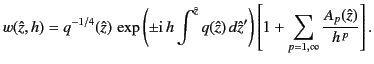(1172)

This is, in fact, an asymptotic series in. We can now appreciate that a WKB solution is just a highly truncated asymptotic series in, in which only the first term in the series is retained.

But, why is it so important that we recognize that WKB solutions are highly truncated asymptotic series? The point is that the WKB method was initially rather controversial after it was popularized in the 1920s. Many scientists thought that the method was not mathematically rigorous. Let us try to understand the origin of the problem. Suppose that we have never heard of an asymptotic series. Looking at Equation (1174), we would imagine that the expression in square brackets is a power law expansion in. The WKB approximation involves neglecting all terms in this expansion except the first. This sounds fine, as long asis much greater than unity. But, surely, to be mathematically rigorous, we have to check that the sum of all of the terms in the expansion that we are neglecting is small compared to the first term? However, if we attempt this then we discover, much to our consternation, that the expansion is divergent. In other words, the sum of all of the neglected terms is infinite! Thus, if we interpret Equation (1174) as a conventional power law expansion inthen the WKB method is clearly nonsense: in fact, the WKB solution would be the first approximation to infinity. However, once we appreciate that Equation (1174) is actually an asymptotic series in, the fact that the series diverges becomes irrelevant. If we retain the first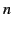terms in the series then the series approximates the exact solution of Equation (1169) with an intrinsic (fractional) error which is of order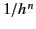(i.e., the first neglected term in the series). The error is minimized at a particular value of. As the number of terms in the series is increased, the intrinsic error decreases, and the value ofat which the error is minimized increases. In particular, we can see that there is an intrinsic error associated with a WKB solution that is of ordertimes the solution.

It is amusing to note that if Equation (1174) were not a divergent series then it would be impossible to obtain total reflection of the WKB solutions at the point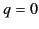. As we shall discover, such reflection is directly associated with the fact that the expansion (1174) exhibits a Stokes phenomenon. It is, of course, impossible for a convergent power series expansion to exhibit a Stokes phenomenon.Next: Stokes Constants Up: Wave Propagation in Inhomogeneous Previous: Asymptotic Series
Richard Fitzpatrick 2014-06-27